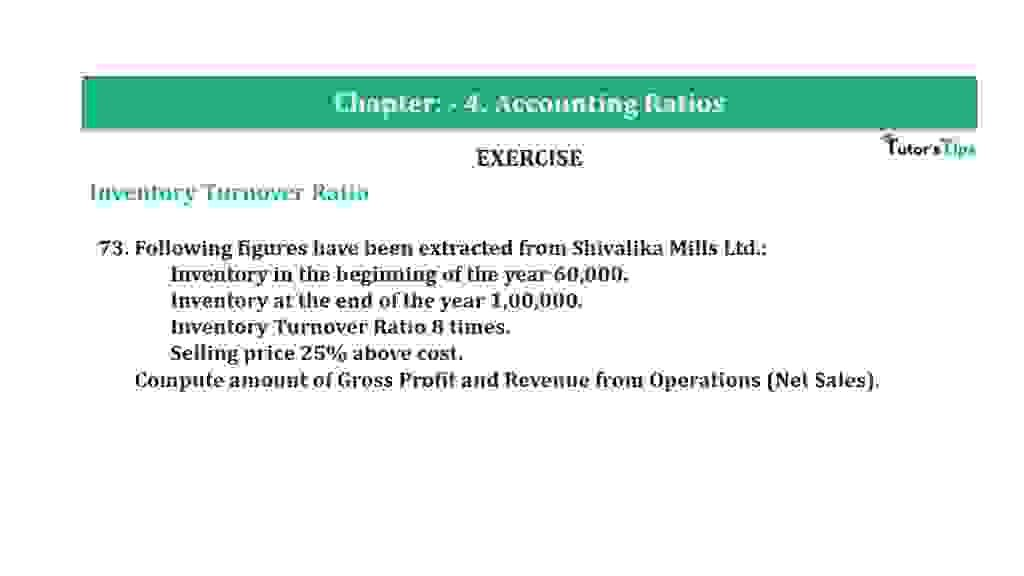# Question 73 Chapter 4 of +2-B – T.S. Grewal 12 ClassQuestion 73 Chapter 4 of +2-B

Interest Coverage Ratio

73. Following figures have been extracted from Shivalika Mills Ltd.:
Inventory in the beginning of the year 60,000.
Inventory at the end of the year 1,00,000.
Inventory Turnover Ratio 8 times.
Selling price 25% above cost.
Compute the amount of Gross Profit and Revenue from Operations (Net Sales).

### The solution of Question 73 Chapter 4 of +2-B: –

 Average Inventory = Opening Inventory + Closing Inventory Average Inventory
 Average Inventory = Rs. 60,000 + Rs. 1,00,000 2 = Rs. 80,000

 Inventory Turnover Ratio = Cost of goods sold Average Inventory
 8 = Cost of goods sold Rs. 80,000

 Cost of goods sold = Rs. 80,000 x 8 Cost of goods sold = Rs. 6,40,000

 Gross Profit = Rs.6,40,000 x 25 100 = Rs.1,60,000

 Sales = Cost of goods sold + Gross Profit = Rs. 6,40,000 + Rs. 1,60,000 = Rs. 8,00,000

Balance Sheet: Meaning, Format & Examples

Thanks, Please Like and share with your friends

Comment if you have any question.

Also, Check out the solved question of previous Chapters: –

### T.S. Grewal’s Double Entry Book Keeping (Vol. II: Accounting for Companies)T.S. Grewal’s Analysis of Financial Statements